# 第六章：概率和朴素贝叶斯

## 朴素贝叶斯### 概率

• 投掷硬币：P(正面) = 0.5
• 掷骰子：P(1) = 1/6
• 青少年：P(女生) = 0.5

P(女生|弗兰克学院的学生) = 0.86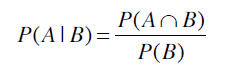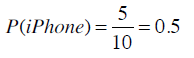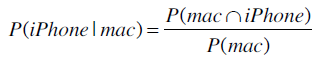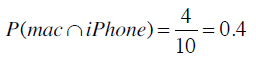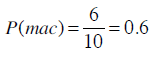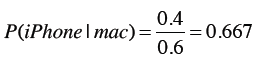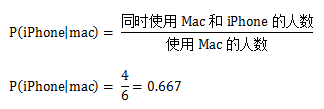iPhone用户中使用Mac的概率是？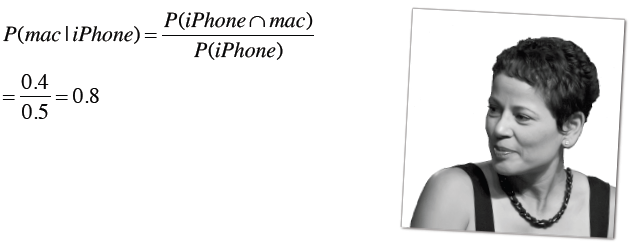P(h)表示事件h发生的概率，称为h的先验概率。在我们进行任何计算之前就已经得知人们使用Mac的概率是0.6。计算之后我们可能会得知使用Mac的人同时会使用iPhone。

P(h|d)称为后验概率，表示在观察了数据集d之后，h事件发生的概率是多少。比如说，我们在观察了使用iPhone的用户后可以得出他们使用Mac的概率是多少。后验概率又称为条件概率

## 微软购物车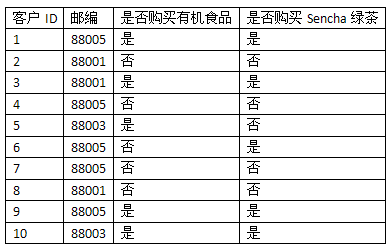P(D)表示从训练集数据中计算得到的概率，比如上表中邮编为88005的概率是：

P(88005) = 0.5

P(D|h)表示在一定条件下的观察结果。比如说购买过Sencha绿茶的人中邮编为88005的概率为：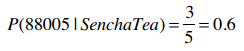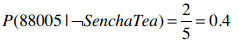P(88001) = 0.3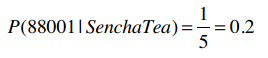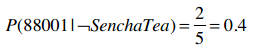## 贝叶斯法则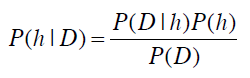• 这位用户会购买Sencha绿茶的概率，即：P(购买|88005)；
• 不会购买的概率：P(┐购买|88005)。

• 居住在88005地区
• 有一个正在读大学的女儿
• 练习瑜伽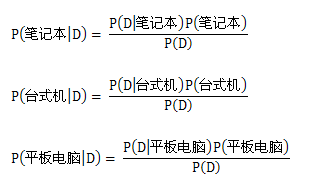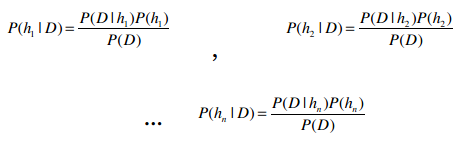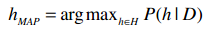H表示所有的事件，所以h∈H表示“对于集合中的每一个事件”。整个公式的含义就是：对于集合中的每一个事件，计算出P(h|D)的值，并取最大的结果。使用贝叶斯法则进行替换：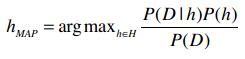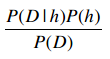• 美国有0.8%的人患有这种癌症：P(癌症) = 0.008
• 99.2%的人没有患有这种癌症：P(┐癌症) = 0.992
• 对于患有癌症的人，他的血液检测结果返回阳性的概率是98%：P(阳性|癌症) = 0.98
• 对于患有癌症的人，检测结果返回阴性的概率是2%：P(阴性|癌症) = 0.02
• 对于没有癌症的人，返回阴性的概率是97%：P(阴性|┐癌症) = 0.97
• 对于没有癌症的人，返回阳性的概率是3%：P(阳性|┐癌症) = 0.03Ann到医院做了血液检测，呈阳性。初看结果并不乐观，毕竟这种血液检测的准确率高达98%。那让我们用贝叶斯法则来计算看看：

• P(阳性|癌症)P(癌症) = 0.98 * 0.008 = 0.0078
• P(阳性|┐癌症)P(┐癌症) = 0.03 * 0.992 = 0.0298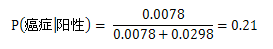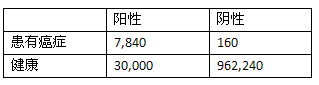Ann的测试结果呈阳性，从上表看阳性中有30,000人其实是健康的，只有7,840人确实患有癌症，所以我们才会认为Ann很有可能是健康的。

## 为什么我们需要贝叶斯法则？P(h1|D) = P(买绿茶|88005)

P(h2|D) = P(┐买绿茶|88005)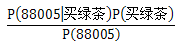P(癌症|阳性) ≈ P(阳性|癌症)P(癌症)

P(┐癌症|阳性) ≈ P(阳性|┐癌症)P(┐癌症)

### 朴素贝叶斯

P(买绿茶|88005 & 买有机食品) = P(88005|买绿茶)P(买有机食品|买绿茶)P(买绿茶) = 0.6 0.8 0.5 = 0.24

P(┐买绿茶|88005 & 买有机食品) = P(88005|┐买绿茶)P(买有机食品|┐买绿茶)P(┐买绿茶) = 0.4 0.25 0.5 = 0.05

## i100、i500健康手环iHealth 100iHealth 500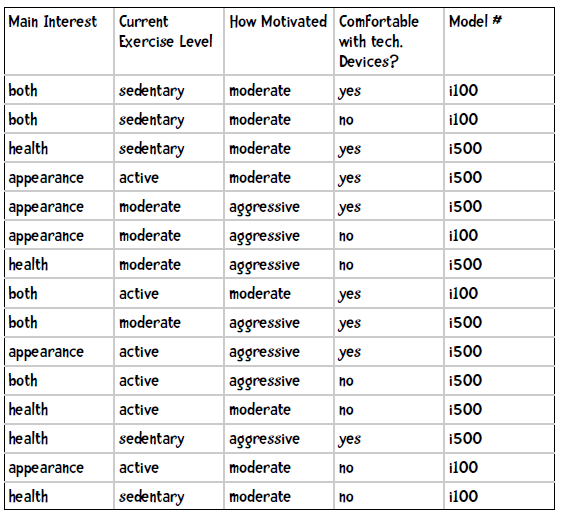``````P(i100|健康，中等水平、热情一般，适应）
P(i500|健康，中等水平，热情一般，适应）
``````

``````P(i100|健康，中等水平、热情一般，适应） = P(健康|i100)P(中等水平|i100)P(热情一般|i100)P(适应|i100)
``````

``````P(健康|i100) = 1/6
P(中等水平|i100) = 1/6
P(热情一般|i100) = 5/6
P(适应|i100) = 2/6
P(i100) = 6/15
``````

``````P(i100|满足条件) = 0.167 * 0.167 * 0.833 * 0.333 * 0.4 = 0.00309
``````

``````P(i500|满足条件) = P(健康|i500)P(中等水平|i500)P(热情一般|i500)P(适应|i500)
= 4/9 * 3/9 * 3/9 * 6/9 * 9/15
= 0.444 * 0.333 * 0.333 * 0.667 * 0.6
= 0.01975
``````

## 使用Python编写朴素贝叶斯分类器

``````both    sedentary    moderate    yes    i100
both    sedentary    moderate    no    i100
health    sedentary    moderate    yes    i500
appearance    active    moderate    yes    i500
appearance    moderate    aggressive    yes    i500
appearance    moderate    aggressive    no    i100
health    moderate    aggressive    no    i500
both    active    moderate    yes    i100
both    moderate    aggressive    yes    i500
appearance    active    aggressive    yes    i500
both    active    aggressive    no    i500
health    active    moderate    no    i500
health    sedentary    aggressive    yes    i500
appearance    active    moderate    no    i100
health    sedentary    moderate    no    i100
``````

### 训练

• 先验概率，如P(i100) = 0.4；
• 条件概率，如P(健康|i100) = 0.167

``````self.prior = {'i500': 0.6, 'i100': 0.4}
``````

``````{'i500': {1: {'appearance': 0.3333333333333333, 'health': 0.4444444444444444,
'both': 0.2222222222222222},
2: {'active': 0.4444444444444444, 'sedentary': 0.2222222222222222,
'moderate': 0.3333333333333333},
3: {'aggressive': 0.6666666666666666, 'moderate': 0.3333333333333333},
4: {'yes': 0.6666666666666666, 'no': 0.3333333333333333}},
'i100': {1: {'both': 0.5, 'health': 0.16666666666666666,
'appearance': 0.3333333333333333},
2: {'active': 0.3333333333333333, 'sedentary': 0.5,
'moderate': 0.16666666666666666},
3: {'aggressive': 0.16666666666666666, 'moderate': 0.8333333333333334},
4: {'yes': 0.3333333333333333, 'no': 0.6666666666666666}}}
``````

1、2、3、4表示第几列，所以第一行可以解释为购买i500的顾客中运动目的是外表的概率是0.333。

``````both    sedentary    moderate    yes    i100
both    sedentary    moderate    no    i100
health    sedentary    moderate    yes    i500
appearance    active    moderate    yes    i500
``````

``````# 第一行
{'i100': 1}

# 第二行
{'i100': 2}

# 第三行
{'i500': 1, 'i100': 2}

# 全部
{'i500': 9, 'i100': 6}
``````

``````{'i100': {1: {'both': 1}}
``````

``````{'i500': {1: {'appearance': 3, 'health': 4, 'both': 2},
2: {'active': 4, 'sedentary': 2, 'moderate': 3},
3: {'aggressive': 6, 'moderate': 3},
4: {'yes': 6, 'no': 3}},
'i100': {1: {'both': 3, 'health': 1, 'appearance': 2},
2: {'active': 2, 'sedentary': 3, 'moderate': 1},
3: {'aggressive': 1, 'moderate': 5},
4: {'yes': 2, 'no': 4}}}
``````

P(外表|i100) = 2 / 6 = 0.333

``````class Classifier:

def __init__(self, bucketPrefix, testBucketNumber, dataFormat):
"""bucketPrefix 分桶数据集文件前缀
testBucketNumber 测试桶编号
dataFormat 数据格式，形如：attr attr attr attr class
"""

total = 0
classes = {}
counts = {}

# 从文件中读取数据
self.format = dataFormat.strip().split('\t')
self.prior = {}
self.conditional = {}
# 遍历十个桶
for i in range(1, 11):
# 跳过测试桶
if i != testBucketNumber:
filename = "%s-%02i" % (bucketPrefix, i)
f = open(filename)
f.close()
for line in lines:
fields = line.strip().split('\t')
ignore = []
vector = []
for i in range(len(fields)):
if self.format[i] == 'num':
vector.append(float(fields[i]))
elif self.format[i] == 'attr':
vector.append(fields[i])
elif self.format[i] == 'comment':
ignore.append(fields[i])
elif self.format[i] == 'class':
category = fields[i]
# 处理该条记录
total += 1
classes.setdefault(category, 0)
counts.setdefault(category, {})
classes[category] += 1
# 处理各个属性
col = 0
for columnValue in vector:
col += 1
counts[category].setdefault(col, {})
counts[category][col].setdefault(columnValue, 0)
counts[category][col][columnValue] += 1

# 计数结束，开始计算概率

# 计算先验概率P(h)
for (category, count) in classes.items():
self.prior[category] = count / total

# 计算条件概率P(h|D)
for (category, columns) in counts.items():
self.conditional.setdefault(category, {})
for (col, valueCounts) in columns.items():
self.conditional[category].setdefault(col, {})
for (attrValue, count) in valueCounts.items():
self.conditional[category][col][attrValue] = (
count / classes[category])
self.tmp =  counts
``````

### 分类

``````c.classify(['health', 'moderate', 'moderate', 'yes'])
````````````    def classify(self, itemVector):
"""返回itemVector所属类别"""
results = []
for (category, prior) in self.prior.items():
prob = prior
col = 1
for attrValue in itemVector:
if not attrValue in self.conditional[category][col]:
# 属性不存在，返回0概率
prob = 0
else:
prob = prob * self.conditional[category][col][attrValue]
col += 1
results.append((prob, category))
# 返回概率最高的结果
return(max(results))
``````

``````>>> c = Classifier('iHealth/i', 10, 'attr\tattr\tattr\tattr\tclass')
>>> c.classify(['health' 'moderate', 'moderate', 'yes'])
i500
``````## 共和党还是民主党

1. 残疾婴儿法案
2. 用水成本分摊
3. 预算改革
4. 医疗费用
5. 萨瓦尔多援助
6. 校园宗教组织
7. 反卫星武器
8. 尼加拉瓜援助
9. MX导弹
10. 移民法案
11. 合成燃料缩减
12. 教育支出法案
13. 有毒废物基金
14. 犯罪
15. 出口免税
16. 南非出口管控

``````democrat    y    n    y    n    n    y    y    y    y    y    n    n    y    n    n    y
democrat    y    y    y    n    n    y    y    y    y    n    n    n    n    n    y    y
republican    y    y    n    y    y    y    n    n    n    y    n    y    y    y    n    n
``````

``````"class\tattr\tattr\tattr\tattr\tattr\tattr\tattr\tattr\tattr\tattr\tattr\tattr\tattr\tattr\tattr\tattr"
``````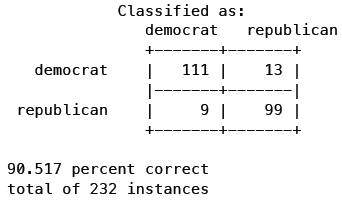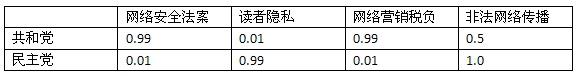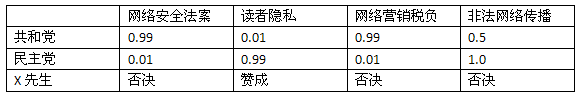``````P(共和党|C=no) = 0.01
P(民主党|C=no) = 0.99
``````

1. 网络安全：C
2. 读者隐私：R
3. 网销税负：T
4. 非法传播：S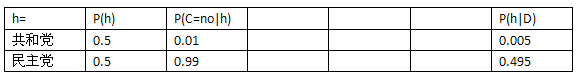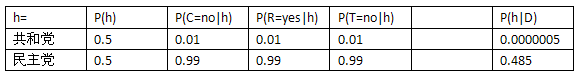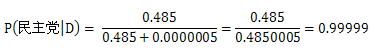### 如何解决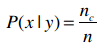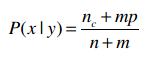m是一个常数，表示等效样本大小。决定常数m的方法有很多，我们这里使用值的类别来作为m，比如投票有赞成和否决两种类别，所以m就为2。p则是相应的先验概率，比如说赞成和否决的概率分别是0.5，那p就是0.5。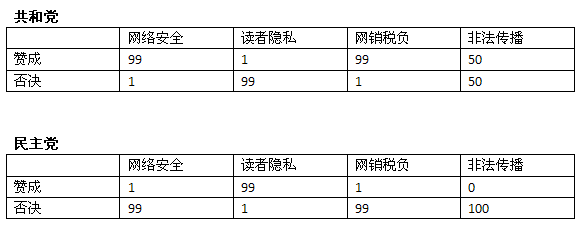X先生对网络安全法案投了否决票，我们先来计算共和党对安全法案投否决票的概率。新的公式是：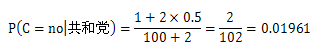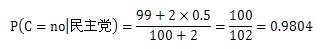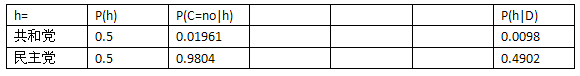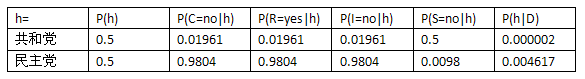### 一点说明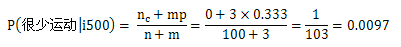## 数值型数据

### 方法一：区分类别

• 年龄
• < 18
• 18 - 22
• 23 - 30
• 31 - 40
• 40

• 年薪
• \$200,000

• 150,000 - 200,000
• 100,000 - 150,000
• 60,000 - 100,000
• 40,000 - 60,000

### 方法二：高斯分布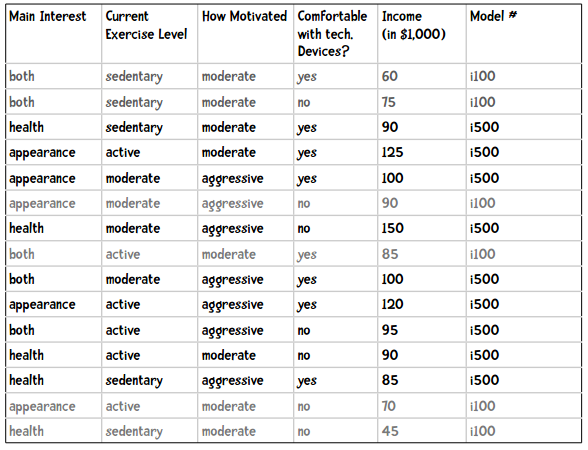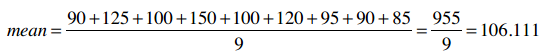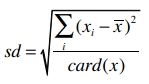### 总体标准差和样本标准差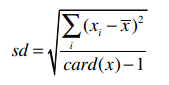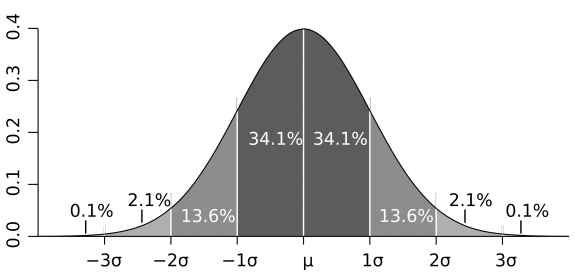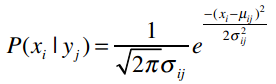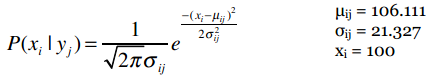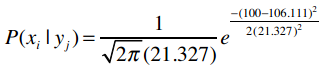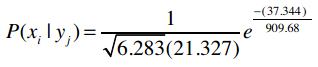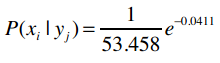e是自然常数，约等于2.718。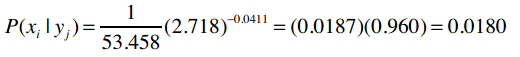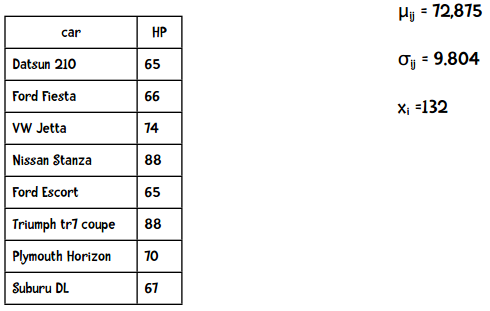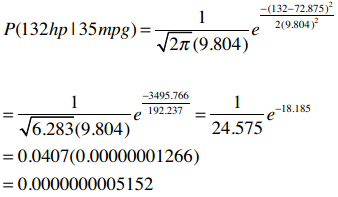### 代码实现提示

``````import math

def pdf(mean, ssd, x):
"""概率密度函数，计算P(x|y)"""
ePart = math.pow(math.e, -(x - mean) ** 2 / (2 * ssd ** 2))
return (1.0 / (math.sqrt(2 * math.pi) * ssd)) * ePart
``````

``````>>> pdf(106.111, 21.327, 100)
0.017953602706962717
>>> pdf(72.875, 9.804, 132)
5.152283971078022e-10
``````## 使用Python实现

### 训练阶段

P(共和党) = 233 / 433 = 0.54

``````{'democrat': {'bill 1': {'yes': 0.333, 'no': 0.667},
'bill 2': {'yes': 0.778, 'moderate': 0.222}},
'republican': {'bill 1': {'yes': 0.811, 'no': 0.189},
'bill 2': {'yes': 0.250, 'no': 0.750}}}
``````

``````mean = {'democrat': {'age': 57, 'years served': 12},
'republican': {'age': 53, 'years served': 7}}
ssd = {'democrat': {'age': 7, 'years served': 3},
'republican': {'age': 5, 'years served': 5}}
``````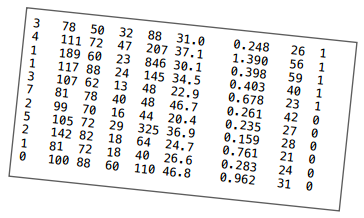• `attr`表示这一列是分类型的特征
• `num` 表示这一列是数值型的特征
• `class` 表示这一列是分类

``````"num    num    num    num    num    num    num    num    class"
``````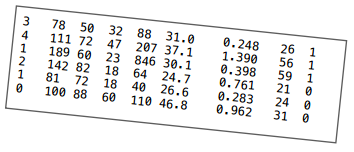``````totals = {'1': {1: 8, 2: 378, 3: 182, 4: 102, 5: 1141,
6: 98.2, 7: 2.036, 8: 141},
'0': {1: 3, 2: 323, 3: 242, 4: 96, 5: 214,
6: 98.1, 7: 2.006, 8: 76}}
``````

``````numericValues = {'1': {1: [3, 4, 1], 2: [78, 111, 189], ...},
'0': {1: [2, 1, 0], 2: [142, 81, 100], ...}}
``````

``````import math

class Classifier:
def __init__(self, bucketPrefix, testBucketNumber, dataFormat):
"""bucketPrefix 分桶数据集文件前缀
testBucketNumber 测试桶编号
dataFormat 数据格式，形如：attr attr attr attr class
"""
total = 0
classes = {}
# 对分类型数据进行计数
counts = {}
# 对数值型数据进行求和
# 我们会使用下面两个变量来计算每个分类各个特征的平均值和样本标准差
totals = {}
numericValues = {}

# 从文件中读取数据
self.format = dataFormat.strip().split('\t')
self.prior = {}
self.conditional = {}

# 遍历1-10号桶
for i in range(1, 11):
# 判断是否跳过
if i != testBucketNumber:
filename = "%s-%02i" % (bucketPrefix, i)
f = open(filename)
f.close()
for line in lines:
fields = line.strip().split('\t')
ignore = []
vector = []
nums = []
for i in range(len(fields)):
if self.format[i] == 'num':
nums.append(float(fields[i]))
elif self.format[i] == 'attr':
vector.append(fields[i])
elif self.format[i] == 'comment':
ignore.append(fields[i])
elif self.format[i] == 'class':
category = fields[i]
# 处理这条记录
total += 1
classes.setdefault(category, 0)
counts.setdefault(category, {})
totals.setdefault(category, {})
numericValues.setdefault(category, {})
classes[category] += 1
# 处理分类型数据
col = 0
for columnValue in vector:
col += 1
counts[category].setdefault(col, {})
counts[category][col].setdefault(columnValue, 0)
counts[category][col][columnValue] += 1
# 处理数值型数据
col = 0
for columnValue in nums:
col += 1
totals[category].setdefault(col, 0)
#totals[category][col].setdefault(columnValue, 0)
totals[category][col] += columnValue
numericValues[category].setdefault(col, [])
numericValues[category][col].append(columnValue)

# 计算先验概率P(h)
for (category, count) in classes.items():
self.prior[category] = count / total

# 计算条件概率P(h|D)
for (category, columns) in counts.items():
self.conditional.setdefault(category, {})
for (col, valueCounts) in columns.items():
self.conditional[category].setdefault(col, {})
for (attrValue, count) in valueCounts.items():
self.conditional[category][col][attrValue] = (
count / classes[category])
self.tmp =  counts

# 计算平均值和样本标准差
self.means = {}
self.ssd = {}
# 动手实践
``````

``````>>> c = Classifier('pimaSmall/pimaSmall', 1, 'num\tnum\tnum\tnum\tnum\tnum\tnum\tnum\tclass')
>>> c.ssd
{'1': {1: 4.21137914295475, 2: 29.52281872377408, ...},
'0': {1: 2.54694671925252, 2: 23.454755259159146, ...}}
>>> c.means
{'1': {1: 5.25, 2: 146.05555555555554, ...},
'0': {1: 2.8867924528301887, 2: 111.90566037735849, ...}}
``````

``````        # 计算平均值和样本标准差
self.means = {}
self.ssd = {}

for (category, columns) in totals.items():
self.means.setdefault(category, {})
for (col, cTotal) in columns.items():
self.means[category][col] = cTotal / classes[category]

for (category, columns) in numericValues.items():
self.ssd.setdefault(category, {})
for (col, values) in columns.items():
SumOfSquareDifferences = 0
theMean = self.means[category][col]
for value in values:
SumOfSquareDifferences += (value - theMean)**2
columns[col] = 0
self.ssd[category][col] = math.sqrt(SumOfSquareDifferences / (classes[category]  - 1))
````````````    def classify(self, itemVector, numVector):
"""返回itemVector所属分类"""
results = []
sqrt2pi = math.sqrt(2 * math.pi)
for (category, prior) in self.prior.items():
prob = prior
col = 1
for attrValue in itemVector:
if not attrValue in self.conditional[category][col]:
# 该特征值没有出现过，因此概率给0
prob = 0
else:
prob = prob * self.conditional[category][col][attrValue]
col += 1
col = 1
for x in  numVector:
mean = self.means[category][col]
ssd = self.ssd[category][col]
ePart = math.pow(math.e, -(x - mean)**2/(2*ssd**2))
prob = prob * ((1.0 / (sqrt2pi*ssd)) * ePart)
col += 1
results.append((prob, category))
# 返回概率最高的分类
#print(results)
return(max(results))
``````

### 朴素贝叶斯的效果要比近邻算法好吗？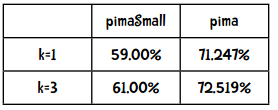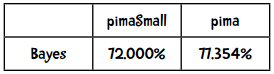kNN算法中，k=3时的Kappa指标是0.35415，效果一般。那朴素贝叶斯的Kappa指标是多少呢？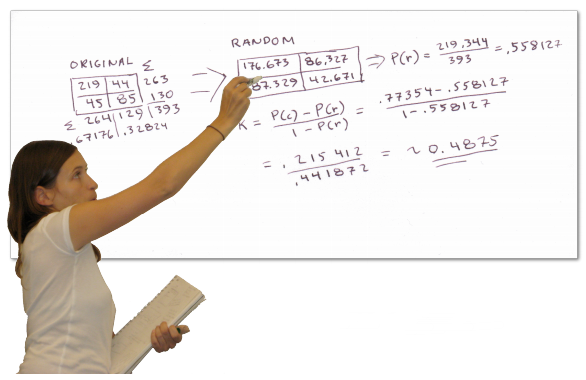• 实现简单（只需计数即可）
• 需要的训练集较少
• 运算效率高

kNN算法的优点：

• 实现也比较简单
• 不需要按特定形式准备数据
• 需要大量内存保存训练集数据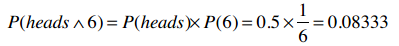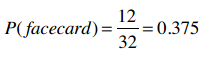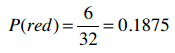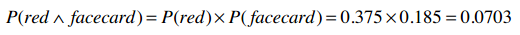• 运动员例子中，身高和体重不是互相独立的，因为高的人体重也会较高。
• 地区邮编、收入、年龄，这些特征也不完全独立，一些地区的房屋都很昂贵，一些地区则只有房车：加州帕罗奥图大多是20岁的年轻人，而亚利桑那州则多是退休人员。
• 在音乐基因工程中，很多特征也是不独立的，如果音乐中有很多变音吉他，那小提琴的概率就降低了。
• 血液检验的结果中，T4和TSH这两个指标通常是呈反比的。

``````>>> tenfold('mpgData/mpgData', 'class\tattr\tnum\tnum\tnum\tnum\tcomment')
``````